Intro to Remainders in a Division Problem Math Video for Kids - Grades 3-5
1%
It was processed successfully!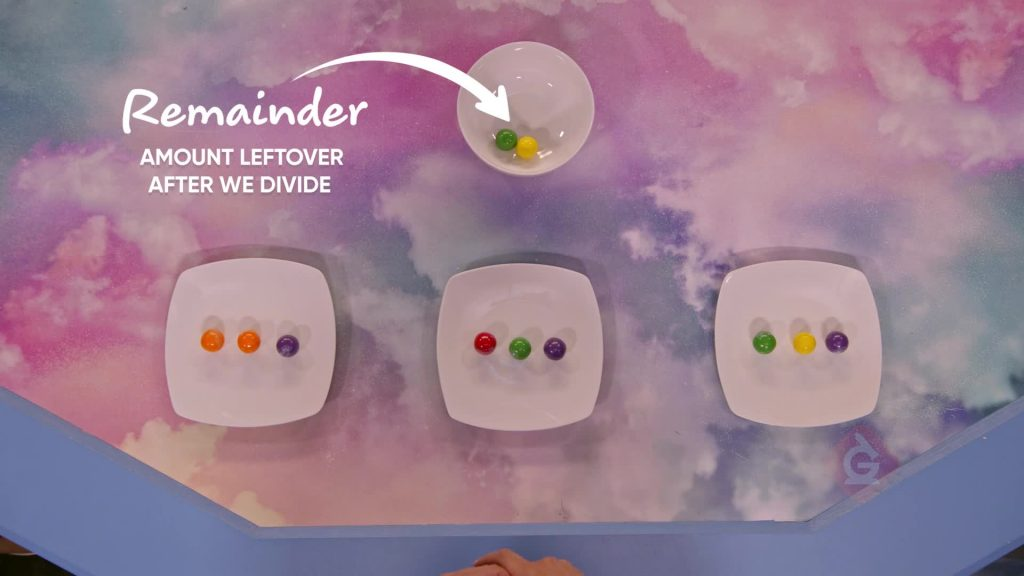WHAT IS A REMAINDER?

Sometimes, when you divide objects into equal-sized groups, there are some left over. This amount is called the remainder. You need to think carefully about how to use the remainder to find answers to different problems.

To better understand remainders in a division problem…

WHAT IS A REMAINDER?. Sometimes, when you divide objects into equal-sized groups, there are some left over. This amount is called the remainder. You need to think carefully about how to use the remainder to find answers to different problems. To better understand remainders in a division problem…

## LET’S BREAK IT DOWN!

### Defining Remainders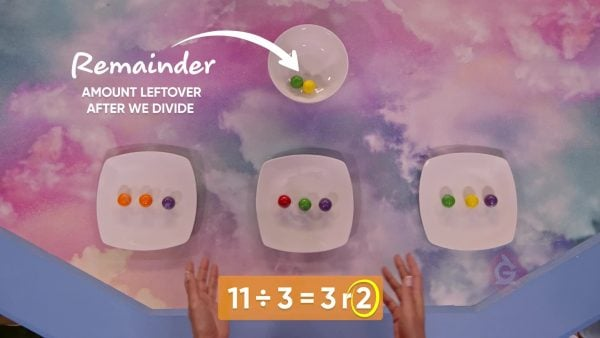If we have 12 gumballs and we want to divide them equally among 3 people, each person gets exactly 4 gumballs. If we have 11 gumballs and we want to divide them equally among 3 people, we only have enough gumballs to give each person 3. We then have 2 gumballs left over, which is not enough to divide equally among 3 people. These 2 gumballs are called the remainder. Then 11 divided by 3 is equal to 3 with a remainder of 2. Now you try: You want to divide 10 gumballs into 3 equal groups. How many does each group get? How many are left over?

Defining Remainders If we have 12 gumballs and we want to divide them equally among 3 people, each person gets exactly 4 gumballs. If we have 11 gumballs and we want to divide them equally among 3 people, we only have enough gumballs to give each person 3. We then have 2 gumballs left over, which is not enough to divide equally among 3 people. These 2 gumballs are called the remainder. Then 11 divided by 3 is equal to 3 with a remainder of 2. Now you try: You want to divide 10 gumballs into 3 equal groups. How many does each group get? How many are left over?

### When can we ignore the remainder?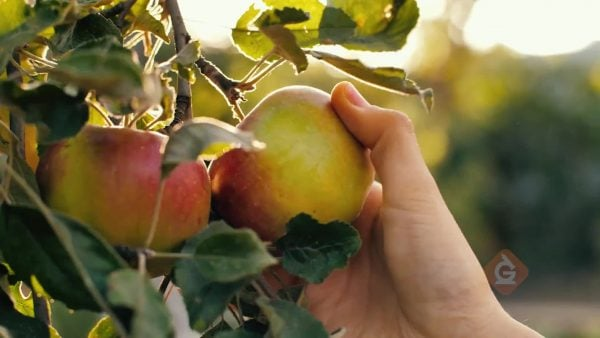You picked 26 apples and you want to make care packages that each contain 4 apples to give to your friends. How many packages of 4 whole apples can you make? We can make 6 groups of 4 apples, and there are 2 apples left over. Since we can’t put parts of apples in the care packages, we can ignore the remainder in this scenario. Now you try: A car fits 3 people. How many cars do you need to fit 17 people? Can you ignore the remainder?

When can we ignore the remainder? You picked 26 apples and you want to make care packages that each contain 4 apples to give to your friends. How many packages of 4 whole apples can you make? We can make 6 groups of 4 apples, and there are 2 apples left over. Since we can’t put parts of apples in the care packages, we can ignore the remainder in this scenario. Now you try: A car fits 3 people. How many cars do you need to fit 17 people? Can you ignore the remainder?

### When do we need to use the remainder?Your whole grade is going to the Air and Space Museum. There are 120 students and teachers going. The school rents buses to transport them. If each bus holds 50 people, how many buses do we need? We need to divide 120 by 50. We can make two groups of 50 from 120, and there are 20 left over. Then 120 ÷ 50 = 2 with a remainder of 20. In this case, we can’t ignore the 20 leftover people. We need to round up and get 3 buses. The third bus won’t be full, but we need to make sure that everyone gets a ride. Now you try: The next grade up has 135 students and teachers going. How many buses would you need?

When do we need to use the remainder? Your whole grade is going to the Air and Space Museum. There are 120 students and teachers going. The school rents buses to transport them. If each bus holds 50 people, how many buses do we need? We need to divide 120 by 50. We can make two groups of 50 from 120, and there are 20 left over. Then 120 ÷ 50 = 2 with a remainder of 20. In this case, we can’t ignore the 20 leftover people. We need to round up and get 3 buses. The third bus won’t be full, but we need to make sure that everyone gets a ride. Now you try: The next grade up has 135 students and teachers going. How many buses would you need?

### When is the remainder the answer?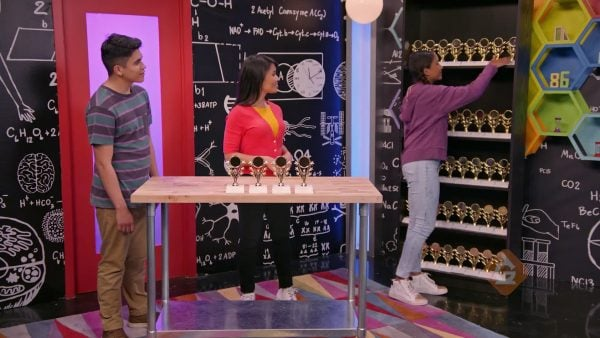You have 5 shelves and you want to display 44 trophies. Each shelf can fit 8 trophies. Are there any trophies that don’t fit on the shelves? We want to solve 44 ÷ 8. We can turn this into a multiplication problem by asking “8 times what number equals 44?” 8 × 6 = 48, which is too much, but 8 × 5 = 40, which is not enough. That means that if we have 5 shelves and each shelf fits 8 trophies, we can fit 40 trophies and we have 4 left over. In this case, the remainder is our answer: 4. Now you try: Sammie bakes a batch of 55 cookies. She puts as many as she can into boxes of 12. How many cookies are left over?

When is the remainder the answer? You have 5 shelves and you want to display 44 trophies. Each shelf can fit 8 trophies. Are there any trophies that don’t fit on the shelves? We want to solve 44 ÷ 8. We can turn this into a multiplication problem by asking “8 times what number equals 44?” 8 × 6 = 48, which is too much, but 8 × 5 = 40, which is not enough. That means that if we have 5 shelves and each shelf fits 8 trophies, we can fit 40 trophies and we have 4 left over. In this case, the remainder is our answer: 4. Now you try: Sammie bakes a batch of 55 cookies. She puts as many as she can into boxes of 12. How many cookies are left over?

### When can we split up the remainder?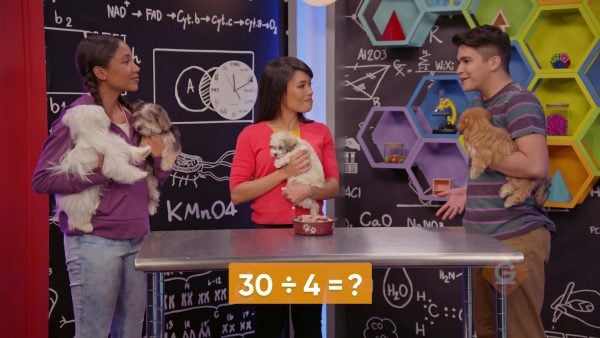You have 30 dog treats that you need to share equally among 4 dogs. To solve this division problem, we can ask, “4 times what is equal to or close to 30?” 4 × 8 = 32, which is too much, because we only have 30 treats. 4 × 7 is 28. That means that 30 divided by 4 is 7 with a remainder of 2. Each dog gets 7 whole treats, and there are 2 left over. In this case, we can split up the remaining 2 treats between 4 dogs using fractions. 4 ÷ = [ggfrac]1/2[/ggfrac], so each dog gets an extra [ggfrac]1/2[/ggfrac] of a treat! Then 30 ÷ 4 = 7[ggfrac]1/2[/ggfrac] with no remainder. Now you try: 14 liters of juice are divided evenly into 3 pitchers. How many liters does each pitcher get?

When can we split up the remainder? You have 30 dog treats that you need to share equally among 4 dogs. To solve this division problem, we can ask, “4 times what is equal to or close to 30?” 4 × 8 = 32, which is too much, because we only have 30 treats. 4 × 7 is 28. That means that 30 divided by 4 is 7 with a remainder of 2. Each dog gets 7 whole treats, and there are 2 left over. In this case, we can split up the remaining 2 treats between 4 dogs using fractions. 4 ÷ = [ggfrac]1/2[/ggfrac], so each dog gets an extra [ggfrac]1/2[/ggfrac] of a treat! Then 30 ÷ 4 = 7[ggfrac]1/2[/ggfrac] with no remainder. Now you try: 14 liters of juice are divided evenly into 3 pitchers. How many liters does each pitcher get?

## INTRO TO REMAINDERS IN A DIVISION PROBLEM VOCABULARY

Divide
To split something up into equal groups.
Dividend
The number being divided.
Divisor
The dividing number.
Quotient
The result of dividing.
Multiply
Remainder
The amount that is left over after something is divided into equal groups.
A number that represents parts of a whole.
When our answer is between two whole numbers and it makes more sense to use the greater number.
When our answer is between two whole numbers and it makes more sense to use the smaller number.

## INTRO TO REMAINDERS IN A DIVISION PROBLEM DISCUSSION QUESTIONS

### Is there a remainder when you divide 15 &#247 3? 15 &#247 4?

15 &#247 3 = 5. There is no remainder. 15 &#247 4 = 3 R 3.

### How can you turn 34 &#247 5 into a multiplication problem?

I want to ask, 5 times what is equal to 34? 5 × 6 = 30 and 5 × 7 = 35, so I know that I have a remainder when I divide 34 &#247 5. If I want to distribute 34 objects evenly into 5 groups, I now know that I can put 6 objects into each group, and then I have 4 left that cannot be distributed. The answer is 6 with a remainder of 4.

### You are sewing dresses and you have 30 meters of fabric. Each dress needs 4 meters. How many dresses can you make?

30 &#247 4 = 7 R 2. I can make 7 dresses, and I have 2 meters of fabric leftover. Maybe I can make a shirt!

### You buy 46 apples at the grocery store and the grocer packs them into boxes for you. Each box fits 10 apples. How many boxes do you need?

46 &#247 10 = 4 R 6. I can fill 4 boxes and then I have 6 apples leftover. I still need a box for those apples, although it will not be full. I need 5 boxes in total.

### There is enough soup in a pot to fill 7 bowls. If 3 people are eating, how much soup does each person get? Is there a remainder or can it all be shared?

7 &#247 3 = 2 R 1. Each person gets 2 bowls of soup, and then there is one bowl left to be shared. The contents of one bowl can be divided by 3, and 1 &#247 3 = [ggfrac]1/3[/ggfrac]. So in total, each person gets 2[ggfrac]1/3[/ggfrac] bowls of soup. Then there is no remainder left!
X

## Success

We’ve sent you an email with instructions how to reset your password.
Ok